Flow measurement can be defined as the quantification of movement of a fluid. The flow measurement is assumed as the oldest recorded work in the instrumentation field. In the industrial field, flow measurement is of great importance as from physiological processes to rocket science, the characteristic of flow is required. Its applications also extend to measurements in day to day processes like gas stations, water service, and so on.

Flow is the third most measured magnitude in industrial processes. It encompasses a wide range of applications from simple ones, like water flow in treatment stations and households, until industrial gases and fuel measurement, in addition to more complex ones. The right choice of a given device in flow measurement depends on many factors. Fluid flow measuring has always been present in our day-to-day work. For example, the residence water meter, the display of a vehicle fuel pump, etc.

Mostly, flow is measured in two ways, volumetric basis and on the basis of weight. The flow of solids is usually measured in terms of mass per unit time or weight per unit time. Liquid flow is measured volumetrically or on the basis of weight. Gaseous flow is normally measured volumetrically. In dealing with flow meters there are two terms known as ‘turn down’ and ‘rangeability’. Turn down is defined as the ratio of full-scale flow to the minimum flow, which can be measured within a stated accuracy. If the turn down is the ratio of 20:1, it means that the flow meter can measure from 20 percent to 100 percent of the scale. This gives the accuracy of the meter. Rangeability is the ratio of maximum to the minimum range to which meter can be calibrated.

## Types of flow meter –

Mechanical type flow meter –
They are the most common and economical type of water flow meters which perform flow measurement through turbine rotation with a shunt, propeller, or paddle wheel design. The mechanical types of water flow meters work by measuring the speed of water flowing through the pipe that causes a piston or turbine to rotate.

Optical flow meters –
Optical flow meters work on the principle of optics i.e. they measure the flow rate using light. Usually, they employ a set-up consisting of a laser beam and photodetectors. Here, the particles of the gas flowing through the pipe scatter the laser beam to produce pulses which are picked-up by the receiver. Then, the time between these signals is determined as one would know the distance by which the photodetectors would be separated, which in turn leads to the measurement of the speed of gas.

Open channel flow meters –
Open channel flow meters are used to measure the flow rate of a fluid whose flow path involves a free surface. Weir meters and Flume meters are the open channel flow meters which use secondary devices like bubblers or float to measure the depth of the fluid at a particular point. From this depth, the flow rate of the fluid can be obtained.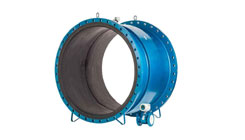Electromagnetic Flow Meter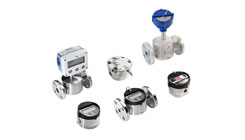Oval Gear Flow Meter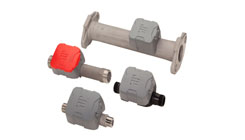Ultrasonic Flow Meter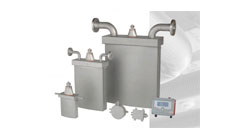Coriolis Flow MeterOpen Channel Flow Meter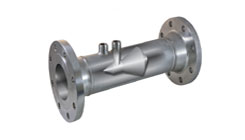Differential Pressure FlowmetersFluid Management System